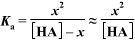# Problem: For many weak acid or weak base calculations, you can use a simplifying assumption to avoid solving quadratic equations. Classify these situations by whether the assumption is valid or the quadratic formula Is required.

###### FREE Expert Solution

1000

94% (26 ratings)###### Problem Details

For many weak acid or weak base calculations, you can use a simplifying assumption to avoid solving quadratic equations.Classify these situations by whether the assumption is valid or the quadratic formula Is required.